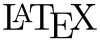# 5 “Tricks” I Wish I Had Known About TeX at the BeginningWe have all had to learn to type using LaTeX. For most, myself included, trying to learn TeX is a nightmare. Although I consider myself nowhere near an expert in LaTeX, I have learned a couple of things the hard way. The following is a list of tips for the beginning (or perhaps experienced) “TeXer.”

1. You can define commands to make it easier to use LaTeX codes that you type regularly. For instance, I use blackboard bold a lot in my papers. Thus, I have defined the command, “newcommand{bb}{mathbb{#1}}”. This allows me to just type bb{R} instead of mathbb{R} to obtain$mathbb{R}$. This may seem like a minor shortcut, but when you use a command frequently, it really helps to have the shortcut.
2. There are great visual editors out there to make your TeX experience easier. There have been posts about some editors before. I do not think TeXMaker has been mentioned though. I have found it to be a very user-friendly tool. The great thing is that it is cross-platform. Mac and Windows users can both access it. Visit http://www.xm1math.net/texmaker/ to download.
3. You can define new “environments.” I often like to color code my notes (e.g. theorems are blue, definitions are yellow, examples are green, etc.). To achieve this easily, I use the color package and define “newtheorem{theorem}{color{Tm}{textbf{underline{Theorem}}}}”. I use this for my theorems, corollaries, claims, definitions, lemmas, exercises, notes, examples, and sidebars. Also, with using the color package, I have defined my own colors by using “definecolor{Tm}{rgb}{0,0,0.80}”. However, the color package has a wealth of predefined colors. See http://en.wikibooks.org/wiki/LaTeX/Colors for a list.
4. Sometimes I want to put equations in an equation environment, but I do not want to number them because I am not going to reference them. If you have an equation that you would like to number, you would have “begin{equation} label{equation1} EQUATION TEX HERE end{equation}”. Note that “equation1” would be whatever you want to use to reference that equation later. Furthermore, to reference the equation later, you would use “$ref{equation1}$”. However when you do not want the equation to be numbered, you would use “begin{equation*} EQUATION TEX HERE end{equation*}”.
5. There is a nifty website that you can draw the symbol you want to use and it will give you the LaTeX code. This cuts down on having to search through documentation to find the code for the symbol you need. The website is http://detexify.kirelabs.org/classify.html.

There is much to learn about LaTeX, but hopefully this helps make it easier. If you have any tips, please share them. I have uploaded part of my Real Analysis notes at http://tylermath.com/latex/RealNotes.pdf. The http://tylermath.com/latex/RealNotes.tex file will demonstrate how to define commands and such.

This entry was posted in Advice, General, Mathematics Online, Technology & Math. Bookmark the permalink.

### 1 Response to 5 “Tricks” I Wish I Had Known About TeX at the Beginning

1.sa says:

In reference to Q4: “equation with no number” surround equation with double dollar sings ($$). Example:$$ sum_{i=1}^{10} = i^2